GRE Subject Test: Math : Infinite Sequences

Example Questions

Example Question #161 : Calculus

Which of the following are not infinite sequences?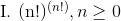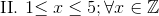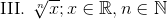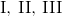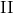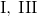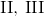Explanation:

Step 1: Define what an infinite sequence is...

An infinite sequence is a sequence that is non-terminating.

Step 2: Determine if each sequence above is infinite...

For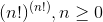, the sequence is always infinite because the set of factorials is infinite. Also, the set of values by raising two factorial powers together is also infinite, it never has an ending term.

For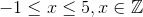, this sequence is FINITE!

For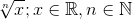, this sequence is FINITE!

All GRE Subject Test: Math Resources